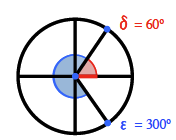### Home > INT3 > Chapter 10 > Lesson 10.3.2 > Problem10-168

10-168.

Without a calculator, what are two solutions to each of the following equations for $0^\circ ≤ θ < 360^\circ$?

1. $\text{cos}(θ) =\frac { 1 } { 2 }$

Draw a unit circle.$\text{The cosine is where the }x\text{-value (red line) is }\frac{1}{2}.$

Answers: $60^\circ$ and $300^\circ$

1. $\text{tan}\left(θ\right) = -1$

$135^\circ$ and $315^\circ$

1. $\text{sin}(θ) =\frac { \sqrt { 3 } } { 2 }$

Draw and label a unit circle as in part (a). Don't forget that the sine is the $y$-value! Which quadrants will the angles be in?

1. $\text{cos}(θ) = -\frac { \sqrt { 3 } } { 2 }$

See part (c).